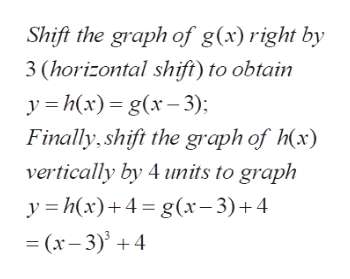# Starting with the graph of a basic​ function, graph the following function using the techniques of​ shifting, compressing,​ stretching, and/or reflecting. Find the domain and range of the function. f(x)=-(x-3)^3+4

Question
10 views
Starting with the graph of a basic​ function, graph the following function using the techniques of​ shifting, compressing,​ stretching, and/or reflecting. Find the domain and range of the function.

f(x)=-(x-3)^3+4
check_circle

Step 1

To determine the graph of the given function, using basic transformations

Step 2

The idea is to build the function (or graph of ) y=f(x) starting with the simpler function (graph) y=x^3, by shifts in the horizontal and vertical directions by  3 and 4 units respectively

Step 3

the required shifts (t...help_outlineImage TranscriptioncloseShift the graph of g(x) right by 3 (horizontal shift) to obtain y h(x g(3) Finally,shift the graph of x) vertically by 4 umits to graph yhx)4g(x-3)+4 = (x-3) + 4 fullscreen

### Want to see the full answer?

See Solution

#### Want to see this answer and more?

Solutions are written by subject experts who are available 24/7. Questions are typically answered within 1 hour.*

See Solution
*Response times may vary by subject and question.
Tagged in

### Algebra